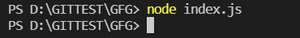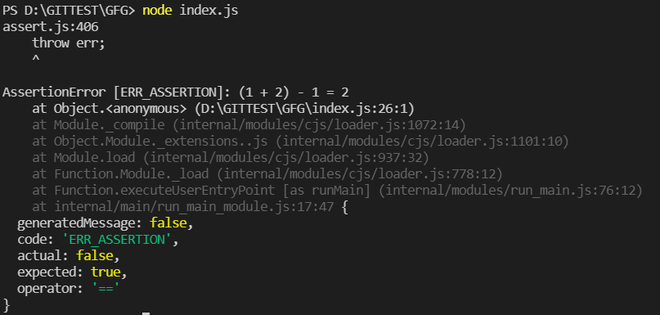Open in App
Not now

# What is the role of assert in Node.js ?

• Last Updated : 08 Nov, 2021

Assert is a Node.js module which provides facilitates to write the test and will not provide any output on the terminal when the test is in the process until any asserting error during the test. It provides various set assertion functions that can be used verifying constants. Assert module in mostly intended for internal use by we can use it in the application.

Role of Assert in Node.js: The role of the assert module provides functions for testing expressions. While testing if any of the expressions evaluates to 0 (false) an assertion failure occurs and the program will be terminated.

Advantages of using the Assert module:

• It helps to minimize the time to debug the code.
• It can be reused across multiple projects if developed with the intent of keeping it in mind.
• It improves error detection.
• It provides better monitoring of the design and helps in easier debug of test failures.
• It can be used for both dynamic simulations as well as in formal verification of the design.

Importing module:

`var assert = require("assert");`

Example 1: The below sample code will not provide any output because the assert case is true.

## index.js

 `// Importing  assert module``var` `assert = require(``'assert'``);  `` ` `function` `expression (a, b, c) {  ``  ``return` `(a + b) - c;  ``} ``// Calling the function``var` `output = expression (1,2,1);  ``assert( output === 2, ``'(1 + 2) - 1 = 2'``);`

Run index.js file using below command:

`node index.js`

OutputExample 2: The below sample code will display an AssertionError as the assert condition is false.

## index.js

 `// Importing the assert module``var` `assert = require(``'assert'``);  `` ` `function` `expression (a, b, c) {  ``  ``return` `(a + b) - c;  ``} ``// Calling the function``var` `output = expression (1,2,1);  ``assert( output === 3, ``'(1 + 2) - 1 = 2'``);`

Run index.js file using below command:

`node index.js`

OutputMy Personal Notes arrow_drop_up